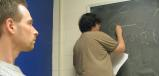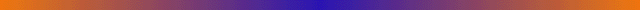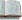next session | course info | google group | description | FAQs | ChaosBook | projects | webbook help | svn | Cvitanović travel schedule ]

CHAOS, AND WHAT TO DO ABOUT ITChaosBook.org study group 2/06 PHYS 8901 PC Spring semester 2006 Mon-Wed 4-5:30pm Howey W505PROBLEM SETS: Problems to be worked will be decided at meetings, and then the solutions presented at the following meeting.

SOLUTIONS: included into ChaosBook.org so far (boyscout version 12, Jan 12 2006)Overture
That deterministic dynamics leads to chaos is no surprise to anyone who has tried pool, billiards or snooker - that is what the game is about - so we start our course about what is chaos and what to do about it by a game of pinball. This might seem a trifle trivial, but a pinball is to chaotic dynamics what a pendulum is to integrable systems: thinking clearly about what is "chaos" in a pinball will help us tackle more difficult problems, such as computing diffusion constants in deterministic gases, or computing the Helium spectrum. We all have an intuitive feeling for what a pinball does as it bounces between the pinball machine disks, and only high school level Euclidean geometry is needed to describe the trajectory. Turning this intuition into calculation will lead us, in clear physically motivated steps, to almost everything one needs to know about deterministic chaos: from unstable dynamical flows, Poincaré sections, Smale horseshoes, symbolic dynamics, pruning, discrete symmetries, periodic orbits, averaging over chaotic sets, evolution operators, dynamical zeta functions, Fredholm determinants, cycle expansions, quantum trace formulas and zeta functions, and to the semiclassical quantization of helium.

Reading:Chapter 1: An overview of the main themes of the course. Recommended reading before you decide to download anything else.Appendix - A brief history of chaos: Classical mechanics has not stood still since Newton. The formalism that we use today was developed by Euler and Lagrange. By the end of the 1800's the three problems that would lead to the notion of chaotic dynamics were already known: the three-body problem, the ergodic hypothesis, and nonlinear oscillators.
e-mail description

Trajectories
We start out by a recapitulation of the basic notions of dynamics. Our aim is narrow; keep the exposition focused on prerequsites to the applications to be developed in this text. I assume that you are familiar with the dynamics on the level of introductory texts such as Strogatz, and concentrate here on developing intuition about what a dynamical system can do. It will be a coarse brush sketch - the full description of all possible behaviors of dynamical systems is anyway beyond human ken.
e-mail description

Reading: Go with the flow(boyscout version 12, Jan 12 2006)Exercises
Reading: Do it again(boyscout version 12, Jan 12 2006)Exercises

Local stability
We continue the discussion of local properties of flows and maps: Henon map, linear stability, types of eigenvalues for linear maps, stable/unstable manifolds.

Reading: Local stability(boyscout version 12, Jan 12 2006)Exercises

Eager to get to the point, we have fast forward to [the boyscout] chapter Cycle Stability:

Newtonian mechanics
Nature is partial to flows invariant with respect to the symplectic structure, that is, flows of Hamiltonian systems. The ability to express mechanical systems in terms of Hamilton's equations provides us with a framework to study general properties and symmetries of this type of flows, and - in particular - study the local stability of equilibria and periodic orbits.

Reading: Newtonian dynamics(boyscout version 12, Jan 12 2006)Exercises
problem set 4

Billiards
Billiards, Bunimovich-Sinai formula for linear stability in billiards.

Reading: Billiards(boyscout version 12, Jan 12 2006)Exercises
Reading: Get straight(boyscout version 12, Jan 12 2006)Exercises

Cycle Stability

In this session we finish discussion of the Poincare return map Jacobian and the full flow Jacobian for the Rossler system, and start discussion of stability of periodic orbits.

Reading: Cycle stability(boyscout version 12, Jan 12 2006)Exercises

Transporting densities
So far we learned how to track an individual trajectory, and its small neighborhood. While the trajectory of an individual representative point may be highly convoluted, the density of these points might evolve in a manner that is relatively smooth. The evolution of the density of representative points is for this reason (and other that will emerge in due course) of great interest.

Reading: Transporting densities(boyscout version 12, Jan 12 2006)
Optional reading: Appendix K: Infinite dimensional operators(boyscout version 12, Jan 12 2006)
Infinite dimensional operators (for students with advanced exposure to quantum mechanics, and mathematically inclined, mostly)Exercises
problem set 5
solutions to problem set 4

Dynamical averaging
In chaotic dynamics detailed prediction is impossible, as any finitely specified initial condition, no matter how precise, will fill out the entire accessible phase space (similarly finitely grained) in finite time. Hence for chaotic dynamics one does not attempt to follow individual trajectories to asymptotic times; what is possible (and sensible) is description of the geometry of the set of possible outcomes, and evaluation of the asymptotic time averages. Examples of such averages are transport coefficients for chaotic dynamical flows, such as the escape rate, mean drift and the diffusion rate; power spectra; and a host of mathematical constructs such as the generalized dimensions, Lyapunov exponents and the Kolmogorov entropy. We shall now set up the formalism for evaluating such averages within the framework of the periodic orbit theory. The key idea is to replace the expectation values of observables by the expectation values of generating functionals. This associates an evolution operator with a given observable, and leads to formulas for its dynamical averages.

Reading: Averaging(boyscout version 12, Jan 12 2006)Exercises
problem set 5

Dynamics, qualitative I
We start learning how to count: qualitative dynamics of simple stretching and mixing flows is used to introduce symbolic dynamics.

Reading: Qualitative dynamics for pedestrians
Robert Gilmore and Marc Lefranc, The Topology of Chaos: Alice in Stretch and Squeezeland, Sects. 2.5 to 2.11(boyscout version 12, Jan 12 2006)Exercises
problem set 8
solutions to problem set 8

Dynamics, qualitative II
We continue learning how to count: qualitative dynamics of Smale horseshoes is used to introduce pruning, finite subshifts, Markov Graphs and transition matrices.

Reading: Qualitative dynamics for cyclists(boyscout version 12, Jan 12 2006)ChaosBook excerpts: Unstable manifolds (unstable version, might update soon)Exercises

Symmetries and dynamics
Dynamics often comes equipped with discrete symmetries, such as reflection and discrete rotation symmetries. Symmetries simplify and improve the cycle expansions in a rather beautiful way.

Reading: Discrete symmetriesSect. 11.6 Symmetries, tilings (boyscout version 12, Jan 12 2006)Sects. 22.1.1, 22.2, 22.3 (boyscout version 12, Jan 12 2006)Sect. 3.8 Periodic lattices (boyscout version 12, Jan 12 2006)Exercises
Discuss pinball exercises 6.1, 2, 3; 8.1, 2; 11.2, 5. Domenico will also address questions that came up last time about hyperbolicity and non-wandering sets in our discussion of the Smale horseshoe and Henon map.

discuss 13.2, 3, and 4. With our current phase on the periodic orbit of presenters, we will have 13.2 Greco, 13.3 Grigo, 13.4 Halcrow.

Counting
We learn how to count: the traces of powers of the transition matrix count admissible cycles, and the largest eigenvalue of the transition matrix yields the topological entropy. The secular determinant of the transition matrix - the Artin-Mazur zeta function - is expressed in terms of the loops of a Markov diagram.

By now we have covered for the first time the whole distance from diagnosing chaotic dynamcs to computing zeta functions. Historically, These topological zeta functions were the inspiration for injecting statistical mechanics into computation of dynamical averages; Ruelle's zeta functions are a weighted generalization of the counting zeta functions.

Reading: Counting(boyscout version 12, Jan 12 2006)Exercises

The reading assignments: Domenico sec. 13.5.1,13.5.4 (counting cycles), Robert sec. 13.6 (topological zeta function for infinite partitions).

Trace formulas
If there is one idea that you should learn about dynamics, it happens in this lecture(s) and it is this: there is a fundamental local - global duality which says that (global) eigenstates are dual to the (local) periodic geodesics. For dynamics on the circle, this is called Fourier analysis; for dynamics on well-tiled manifolds this is called Selberg trace formulas and zeta functions; and for generic nonlinear dynamical systems the duality is embodied in trace formulas, zeta functions and spectral determinants that we will now introduce. These objects are to dynamics what partition functions are to statistical mechanics. The bold claim is that once you understand this, classical ergodicity, wave mechanics and stochastic mechanics are but special cases, to be worked out at your leasure.

The strategy is this: Global averages such as escape rates can be extracted from the eigenvalues of evolution operators. The eigenvalues are given by the zeros of appropriate determinants. One way to evaluate determinants is to expand them in terms of traces, log det = tr log. The traces are evaluated as integrals over Dirac delta functions, and in this way the spectra of evolution operators become related to periodic orbits.

The rest of the course is making sense out of this objects and learning how to apply them to evaluation of physically measurable properties of chaotic dynamical systems.

Reading: Trace formulas(boyscout version 12, Jan 12 2006)Exercises

The reading assignments: Vaggelis sec. 14.1 (trace of an evolution operator), Ed (for John W.) sec. 14.2 (trace formula for maps), Ed sec. 14.2.1, 14.3 (a trace formula for transfer operators, a trace formula for flows), Alex sec. 14.4 (an asymptotic trace formula), Jonathan sec. 15.1 (spectral determinants for maps), Domenico sec. 15.2 (spectral determinants for flows).

Reading: Spectral determinants(boyscout version 12, Jan 12 2006)Exercises

The reading assignments: Robert sec. 15.3 (dynamical zeta functions), Vaggelis sec. 15.3.2, 15.4 (zeta function for transfer operators, false zeros), John sec. 15.5 (spectral determinants vs. dynamical zeta functions).

Sec. 15.3.1 is on intermittency, we skipped it. Vaggelis sec. 15.3.2, 15.4 (zeta function for transfer operators, false zeros), John sec. 15.5 (spectral determinants vs. dynamical zeta functions), Ed sec. 15.6, 15.7 ("all too many eigenvalues?", equilibrium points)

Eager to get to the point, we have fast forward to [the boyscout] chapter on cycle expansions:

Why does it work?  I
The heuristic manipulations that led to the trace formulas and spectral determinants are potentially dangerous, as we are dealing with infinite-dimensional vector spaces and singular integral kernels. Intuitively, the theory should converge because long cycles are shadowed by nearby pseudo-cycles.

For clasess of not althogether too idealized smooth flows very strong results exists. We explain the ideas behind proofs of Ruelle and Rugh which establish that for nice real analytic expanding or hyperbolic flows the spectral (Fredholm) determinants are entire, and that in this context the edifice constructed in this course has a firm mathematical foundations.

Reading: Why does it work?(boyscout version 12, Jan 12 2006)Exercises

Cycle expansions
So far we have derived a plethora of periodic orbit trace formulas, spectral determinants and zeta functions. Now we learn how to expanded these as cycle expansions, series ordered by increasing topological cycle length, and evaluate average quantites like escape rates. These formulas are exact, and, when the winds are kind, highly convergent. The pleasant surprise is that the terms in such expansions fall off exponentially or even faster, so that a handful of shortest orbits suffices for rather accurate estimates of asymptotic averages.

Reading: Cycle expansions(boyscout version 12, Jan 12 2006)Exercises

The schedule: Alex sec. 18.1, 18.1.1; Jonathan sec. 18.1.2, 18.1.3; Domenico sec. 18.2, 18.2.1; Robert sec 18.2.3, 18.2.4. That's all we have to do for chapter 18.

From deterministic chaos to nonequilibrium statistical mechanics
Back to the meat of the subject; can one derive statistical mechanics from deterministic chaos? We apply the periodic orbit theory to evaluation of measurable properties of a very simple model of ideal gas, periodic Lorentz gas, and derive closed-form formulas for the diffusion constant.

Can one one go beyond equilibrium statistical mechanics and derive properties of systems far from equilibrium by the methods discussed in this course? This is currently a very lively research area, and we explain how the periodic orbit theory yields transport properties in models of dissipative driven systems, such as Guassian thermostatted Lorentz gas.

Reading: Deterministic diffusion(boyscout version 12, Jan 12 2006)Exercises

The schedule: Robert: sec. 23.1; Vaggelis: sec. 23.1.1; Jonathan : sec. 23.2; Alex: sec. 23.2.1. After that we should be able to calculate the diffusion coefficient for a chain of piecewise linear maps, the simplest case of 1d scattering.

Lat meeting Monday May 1, at 3pm: section 23.2.3 on diffusion for finite Markov partitions.Abandon all hope ye who read beyond here, since the rest has yet to be worked out.

``` 	 2006
April         May
Mo Tu We    Mo Tu We Th
1  2  3  4
17 18 19
24 25 26

```

Friday Apr 29:    classes end

grades deadline            Mon May 9Predrag.Cvitanovic at physics.gatech.edusvn: \$Author: predrag \$ - \$Date: 2006-06-26 10:17:00 -0400 (Mon, 26 Jun 2006) \$